## Articulo

•Citado por SciELO
•Accesos

•Similares en SciELO

## versión On-line ISSN 1390-6542versión impresa ISSN 1390-9363

### Enfoque UTE vol.9 no.4 Quito oct./dic. 2018

#### http://dx.doi.org/10.29019/enfoqueute.v9n4.398

Categoria

Fractional Order Modeling of a Nonlinear Electromechanical System

Modelamiento de orden fraccional de un sistema no lineal electromecánico

1 School of Mathematics, Universidad Nacional de Colombia, Medellín - Colombia jerendonr@unal.edu.co, cemejia@unal.edu.co.

Abstract:

This paper presents a novel modeling technique for a VTOL electromechanical nonlinear dynamical system, based on fractional order derivatives. The proposed method evaluates the possible fractional differential equations of the electromechanical system model by a comparison against actual measurements and in order to estimate the optimal fractional parameters for the differential operators of the model, an extended Kalman filter was implemented. The main advantages of the fractional model over the classical model are the simultaneous representation of the nonlinear slow dynamics of the system due to the mechanical components and the nonlinear fast dynamics of the electrical components.

Keywords: Fractional Calculus; Dynamical Electromechanical System; Fractional Parameters Estimation; Extended Kalman Filter.

Resumen:

Este artículo presenta una novedosa técnica de modelamiento dinámico no lineal, basada en derivadas de orden fraccional, para un sistema electromecánico de tipo VTOL. El método propuesto estudia la posibilidad de modelar dinámicamente el sistema electromecánico mediante ecuaciones diferenciales de carácter fraccional realizando una comparación con mediciones reales, de tal forma que con base en estas mediciones y un filtro de Kalman extendido, nosotros logremos estimar los parámetros fraccionales óptimos para los operadores diferenciales fraccionales. La ventaja principal del modelamiento fraccional con respecto al modelamiento clásico, radica en que el primero logra representar mejor las diferentes dinámicas lentas y rápidas presentes en los sistemas electromecánicos debidas a las componentes mecánicas y eléctricas respectivamente.

Palabras clave: Cálculo Fraccional; Sistema Dinámico Electromecánico; Estimación de Parámetros Fraccionales; Filtro de Kalman Extendido.

1. Introduction

Fractional calculus is a field of mathematical analysis in which integro-differential operators of arbitrary order have an essential role; this field goes back to the times of Leibniz, around 1695, but in the last few decades it has become a very active research topic. Some of the current applications are in viscoelastic materials, heat transfer and diffusion, wave propagation, electrical circuits, electromagnetic theory, modeling and control of dynamical systems to mention only a few (Miller and Ross, 1993; Tenreiro et al., 2010; Rahimy, 2010).

This research focuses on the modeling of electromechanical systems by fractional derivatives. It is motivated and somewhat related to the works by several authors, namely:

(Gómez et al., 2016): The authors present an analytical and numerical study of the differential equations for an RLC electric circuits dynamical model by fractional derivatives. The considered system does not include mechanical components and there are no actual measurements.

(Chen et al., 2017): This reference introduces a design for DC-DC converters based on fractional order derivatives. There are comparisons with actual frequency measurements.

(Schäfer and Krüger, 2006): The behavior of an iron core with saturation is evaluated based on changes in the frequency of the electromagnetic field, behavioral data is saved and used to design a fractional core circuit model. Although in this study actual measurements are used, there are no mechanical components in the system and the fractional parameters are not fully explained.

(Özkan, 2014) and (Swain et al., 2017): These references propose fractional PID controls for electromechanical systems with integer order models. As proposed by (Podlubny, 1999), it is important to look for an alternative fractional derivatives model, since the proposed controls exhibit poor performance.

(Lazarevi et al., 2016) and (Petrás, 2011): They show the direct relationship between modeling based on fractional derivatives and duality mechanical-electrical of electromechanical systems. The model identification is made in frequency domain.

The aim of this paper is the modeling of a dynamical electromechanical system by fractional differential equations. Furthermore, the fractional orders of the derivatives are optimal and are estimated by an extended Kalman filter (EKF), based on actual time measurements. As far as we know, this approach is new and worthy.

The paper is organized as follows: Section 2 introduces the fractional derivatives by Grünwald-Letnikov and Caputo, the dynamical models for a VTOL electromechanical system and the EKF estimation procedure. The numerical results are the subject of Section 3 and Section 4 is devoted to the conclusions.

2. Methodology

Firstly, we introduce the fractional order derivatives in the sense of Grünwald-Letnikov and Caputo. Subsequently we consider the mathematical model for the VTOL electromechanical system and the method of parameter estimation known as extended Kalman filter.

2.1 Definitions of fractional order derivatives

In the development of the fractional calculus several definitions for the arbitrary order derivatives and integrals have appeared. The best known are: the Grünwald-Letnikov (GL), the Caputo (C) and the Riemann-Liouville (RL) definitions. The first two are the ones implemented in this work (Podlubny, 1998).

2.1.1 Grünwald - Letnikov derivative

Let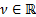. The Grünwald-Letnikov fractional derivative of order ν of a real function f is basically an extension of the backward finite difference formula (Swain et al., 2017). It is given by

where nh = t - a.

This definition can be easily approximated by taking a discretization parameter h small enough. The numerical approximation is given by

where m - 1 < ν < m.

2.1.2 Caputo derivative

Let. The Caputo fractional derivative of order ν of a real function f is given by

where m - 1 < ν < m.

As stated in (Li and Ma, 2013) and (Podlubny, 1998) the Caputo initial value problem

is equivalent to the Volterra integral equation of the second kind, given by

The Caputo derivative can be approximated by a quadrature rule like a trapezoidal rule, i.e.

Now we proceed to the modeling phase of our work.

2.2 Modeling of an electromechanical system

A Qnet vertical take-off and landing (VTOL) is the electromechanical system considered here. This system consists on a speed fan with helices (motor actuator) adjusted to a fixed base by one arm and a counterweight on the other side as it is shown in Figure 1. The air flow through the propellers affects the dynamics of the system, and changes the angular position of the weight by the rotation of the arm around the pivot. Some applications of this system in real world devices are helicopters, rockets, balloons, and harrier jets (Apkarian et al., 2011).

2.2.1 Physics of the system

The mechanical, electrical and aerodynamic laws that explain the behavior of the system, allow us to characterize the nonlinear dynamical model of the system by the following set of equations:

This set of equations is denoted (NLS). In search of a nearby simpler set of equations, (Apkarian et al., 2011) propose a simplification for the first and the third equations given by

and Ohm's Law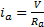respectively.

The modification of the first equation corresponds to the replacement of the thrust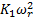and the drag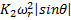by the standard torque motor relation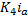.

Based on this work, we propose a simplified system of equations which is nonlinear. It maintains the gravitational torque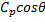in the first equation and takes into account the counter-electromotive torque in the arm and rotor angular velocities. The new simplified nonlinear system, denoted (SNLS), is

The variables and parameters are described in Tables 1 and Table 2 which were identified by using the methodology proposed in (Apkarian et al., 2011). Since the actual measurements of the system are for the variables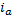and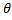, then our analysis is based on these two variables.

The estimation of the fractional orders of derivatives of the model is made by an extended Kalman Filter (EKF) procedure for which we follow (Sierociuk and Dzieliski, 2006); here a fractional order parameter estimation is proposed by the authors, this methodology is based on the Grünwald-Letnikov definition and uses an EKF structure which is described below.

2.2 EKF structure estimation

Consider the following discrete nonlinear system:

where: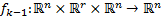is a discrete vector field that relates the system state variables at instant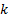, with themselves and the uncertainty of modeling at instant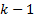.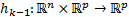is a discrete vector field that relates the system state variables with the output variables.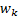and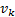represent modeling uncertainty and measurement noise respectively.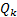and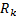are the matrices of variance in modeling uncertainty and measurement noise respectively.

There are a prediction step and a correction step denoted by the indexes () and (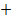) respectively. First the initial conditions for the EKF are determined by the following equations where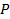is the covariance matrix of the states.

Let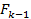be the Jacobian matrix of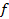at time step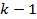. Thus, the prediction step is given by

Finally, the correction step or state estimation is given by

It should be noted that the state estimation requires system measurements and an idea of the noise variance present in these measurements and in the model.

3. Results and discussion

In Figures 2andFigure 3, a time step response of the three models are shown, this simulation was done by applying Euler method with step size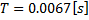, and an input voltage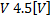, which are the same step size and voltage of the actual measurements, which are also shown in the figures. The best agreement with experimental results is obtained by the simplified nonlinear model (SNLS) and Table 3 is a short summary of this fact.

3.1 Fractional model for the system

A fractional version of the simplified nonlinear model is denoted (FSNLS) and is given by

To evaluate the performance of the new model a thorough sensitivity analysis is implemented. The unknown parameters are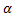,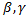and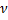in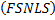which are allowed to take values in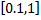. One of the main observations is that if the first and third equations in (FSNLS) had fractional derivatives, the matching with measurements is optimal. Figures 4 and Figure 5 illustrate this fact.

Based on a sensitivity analysis reported in (Rendón, 2018), the fractional model is given by (FSNLS) with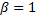and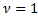. That is, the second and fourth equations are not fractional. The selection of the fractional derivative orders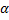and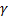is made by an extended Kalman filter procedure described below.

3.2 Parameter Estimation

In (Sierociuk and Dzieliski, 2006) it is shown that the EKF structure is strongly coupled to the fractional order parameters of the GL definition. The additional terms in the definition are considered as modeling noise and the covariance matrix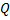is modified by a neural network. We take a different strategy given below. It consists on a discrete redefinition of model (FSNLS) changing GL derivate definition to Caputo derivative definition and parametersandare included as unknown parameters of the system.

If we also have the following measured variables and matrix variances of measurement and modeling

then it is possible to estimate the fractional parametersandbased on EKF methodology. Figure 6 illustrates this point. No attempt on the identification of fractional orders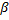andwas made due to the results of the sensitivity analysis. Notice that the choices ofand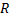are based on the scaling of the measurements and some knowledge on the measurements and model noise variation.

Figure 6 exhibits stationary behavior for parameterand a less stationary trajectory for. Several fractional orders can be selected according to our results. Our pick is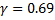and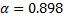wich are among the best considered.

Table 4 and Figure 7 summarize the results. They indicate that the fractional model with GL derivative is an improvement over the classical model for this electromechanical device. We believe our claim can be verified by other researchers. Notice that the fractional model detects the initial current overshoot and none of the classical models introduced above are able to do that.

4. Conclusions

In this paper a new methodology for fractional modeling of a dynamical electromechanical nonlinear system is proposed. As a result, it turns out that fractional order derivatives in two of the four equations of system (FSNLS) provide an improvement over classical models. We reach this conclusion by considering actual measurements and a fractional parameter estimation based on an extended Kalman Filter (EKF) process.

Acknowledgements

The authors would like to thank professor Mónica Ayde Vallejo Velásquez, director of Electronics and Control Laboratory at Universidad Nacional de Colombia for allowing us to take measurements of the VTOL electromechanical system.

The authors acknowledge the support provided by Universidad Nacional de Colombia through the research project Non local operators and operators with memory, Hermes code number 33154.

References

Apkarian, J., Karam, P., Lévis, M., and Martin, P. (2011). “STUDENT WORKBOOK QNET VTOL Trainer for NI ELVIS”. [ Links ]

Chen, X., Chen, Y., Zhang, B., and Qiu, D. (2017). A Modeling and Analysis Method for Fractional-Order DC-DC Converters. IEEE Transactions on Power Electronics, 32(9), 7034-7044. [ Links ]

Gómez, J.F., Yépez, H., Escobar, R.F., Astorga, C.M., and Reyes, J. (2016). Analytical and numerical solutions of electrical circuits described by fractional derivatives. Applied Mathematical Modelling, 40(21), 9079-9094. [ Links ]

Lazarevi, M.P., Mandi, P.D., Cvetkovi, B., ekara, T.B., and Lutovac, B. (2016). Some electromechanical systems and analogies of mem-systems integer and fractional order. In 2016 5th Mediterranean Conference on Embedded Computing (MECO), 230-233. [ Links ]

Li, C. and Ma, Y. (2013). Fractional dynamical system and its linearization theorem. Nonlinear Dynamics, 71(4), 621-633. [ Links ]

Miller, K.s. and Ross, B. (1993). An introduction to the fractional calculus and fractional differential equations. Wiley-Interscience, 1 edition. [ Links ]

Özkan, B. (2014). Control of an Electromechanical Control Actuation System Using a Fractional Order Proportional, Integral, and Derivative-Type Controller. IFAC Proceedings Volumes, 47(3), 4493-4498. [ Links ]

Petrás, I. (2011). Fractional-Order Nonlinear Systems. Nonlinear Physical Science. Springer Berlin Heidelberg, Berlin, Heidelberg. [ Links ]

Podlubny, I. (1999). Fractional-order systems and PIλ Dμ-controllers. IEEE Transactions on Automatic Control, 44(1), 208-214. [ Links ]

Podlubny, I. (1998). Fractional Differential Equations: An Introduction to Fractional Derivatives, Fractional Differential Equations, to Methods of Their Solution and Some of Their Applications. Academic Press. [ Links ]

Rahimy, M. (2010). Applications of fractional differential equations. Applied Mathematica Sciences 4(50): 2453-2461. [ Links ]

Rendón, J. (2018). Aplicaciones del cálculo fraccional en modelamiento y control de sistemas dinámicos electromecánicos. Master's thesis, Universidad Nacional de Colombia, Medellín, Colombia. [ Links ]

Schäfer, I. and Krüger, K. (2006). Modelling of coils using fractional derivatives. Journal of Magnetism and Magnetic Materials, 307(1), 91-98. [ Links ]

Sierociuk, D. and Dzieliski, A. (2006). Fractional kalman filter algorithm for the states parameters and order of fractional system estimation. International Journal of Applied Mathematics and Computer Science, 16(1), 129-140. [ Links ]

Swain, S.K., Sain, D., Mishra, S.K., and Ghosh, S. (2017). Real time implementation of fractional order PID controllers for a magnetic levitation plant. AEU - International Journal of Electronics and Communications, 78, 141-156. [ Links ]

Tenreiro, J.A., Silva, M.F., Barbosa, R.S., Jesus, I.S., Reis, C.M., Marcos, M.G., and Galhano, A.F. (2010). Some Applications of Fractional Calculus in Engineering. Mathematical Problems in Engineeringhttp://dx.doi.org/10.1155/2010/639801Links ]

Received: May 15, 2018; Accepted: October 31, 2018This is an open-access article distributed under the terms of the Creative Commons Attribution License